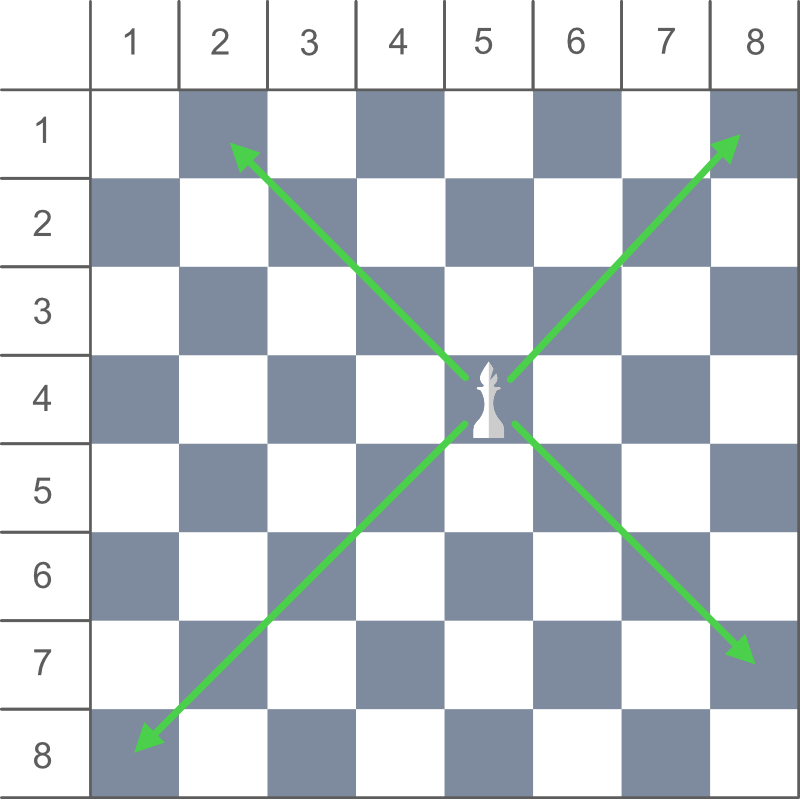# Count the total number of squares that can be visited by Bishop in one move

Given the position of a Bishop on an 8 * 8 chessboard, the task is to count the total number of squares that can be visited by the Bishop in one move. The position of the Bishop is denoted using row and column number of the chessboard.

Examples:

Input: Row = 4, Column = 4
Output: 13

Input: Row = 1, Column = 1
Output:

Approach: In the game of chess, a Bishop can only move diagonally and there is no restriction in distance for each move.So, We can also say that Bishop can move in four ways i.e. diagonally top left, top right, bottom left and bottom right from current position.

We can calculate the numbers of squares visited in each move by:

Total squares visited in Top Left move = min(r, c) – 1
Total squares visited in Top Right move = min(r, 9 – c) – 1
Total squares visited in Bottom Left move = 8 – max(r, 9 – c)
Total squares visited in Bottom Right move = 8 – max(r, c)
where, r and c are the coordinates of the current position of the Bishop on the chessboard.

Below is the implementation of the above approach:

## C++

 `// C++ implementation of above approach` `#include ` `using` `namespace` `std;`   `// Function to return the count of` `// total positions the Bishop` `// can visit in a single move` `int` `countSquares(``int` `row, ``int` `column)` `{`   `    ``// Count top left squares` `    ``int` `topLeft = min(row, column) - 1;`   `    ``// Count bottom right squares` `    ``int` `bottomRight = 8 - max(row, column);`   `    ``// Count top right squares` `    ``int` `topRight = min(row, 9 - column) - 1;`   `    ``// Count bottom left squares` `    ``int` `bottomLeft = 8 - max(row, 9 - column);`   `    ``// Return total count` `    ``return` `(topLeft + topRight + bottomRight + bottomLeft);` `}`   `// Driver code` `int` `main()` `{`   `    ``// Bishop's Position` `    ``int` `row = 4, column = 4;`   `    ``cout << countSquares(row, column);`   `    ``return` `0;` `}`

## Java

 `// Java implementation of above approach` `class` `GFG {`   `    ``// Function to return the count of` `    ``// total positions the Bishop` `    ``// can visit in a single move` `    ``static` `int` `countSquares(``int` `row, ``int` `column)` `    ``{`   `        ``// Count top left squares` `        ``int` `topLeft = Math.min(row, column) - ``1``;`   `        ``// Count bottom right squares` `        ``int` `bottomRight = ``8` `- Math.max(row, column);`   `        ``// Count top right squares` `        ``int` `topRight = Math.min(row, ``9` `- column) - ``1``;`   `        ``// Count bottom left squares` `        ``int` `bottomLeft = ``8` `- Math.max(row, ``9` `- column);`   `        ``// Return total count` `        ``return` `(topLeft + topRight + bottomRight + bottomLeft);` `    ``}`   `    ``// Driver code` `    ``public` `static` `void` `main(String[] args)` `    ``{`   `        ``// Bishop's Position` `        ``int` `row = ``4``, column = ``4``;`   `        ``System.out.println(countSquares(row, column));` `    ``}` `}`

## C#

 `// C# implementation of above approach` `using` `System;` `class` `GFG {`   `    ``// Function to return the count of` `    ``// total positions the Bishop` `    ``// can visit in a single move` `    ``static` `int` `countSquares(``int` `row, ``int` `column)` `    ``{`   `        ``// Count top left squares` `        ``int` `topLeft = Math.Min(row, column) - 1;`   `        ``// Count bottom right squares` `        ``int` `bottomRight = 8 - Math.Max(row, column);`   `        ``// Count top right squares` `        ``int` `topRight = Math.Min(row, 9 - column) - 1;`   `        ``// Count bottom left squares` `        ``int` `bottomLeft = 8 - Math.Max(row, 9 - column);`   `        ``// Return total count` `        ``return` `(topLeft + topRight + bottomRight + bottomLeft);` `    ``}`   `    ``// Driver code` `    ``public` `static` `void` `Main()` `    ``{`   `        ``// Bishop's Position` `        ``int` `row = 4, column = 4;`   `        ``Console.WriteLine(countSquares(row, column));` `    ``}` `}`

## Python3

 `# Python3 implementation of above approach`   `# Function to return the count of ` `# total positions the Bishop ` `# can visit in a single move` `def` `countSquares(row, column):` `    `  `    ``# Count top left squares` `    ``topLeft ``=` `min``(row, column) ``-` `1` `    `  `    ``# Count bottom right squares` `    ``bottomRight ``=` `8` `-` `max``(row, column)` `    `  `    ``# Count top right squares` `    ``topRight ``=` `min``(row, ``9``-``column) ``-``1` `    `  `    ``# Count bottom left squares` `    ``bottomLeft ``=` `8` `-` `max``(row, ``9``-``column) `   `    `  `    ``# Return total count` `    ``return` `(topLeft ``+` `topRight ``+` `bottomRight ``+` `bottomLeft)`   `# Driver code`   `# Bishop's Position ` `row ``=` `4` `column ``=` `4`   `print``(countSquares(row, column))`

## PHP

 ``

## Javascript

 ``

Output

`13`

Complexity Analysis:

• Time Complexity: O(1)
• Auxiliary Space: O(1)

Feeling lost in the world of random DSA topics, wasting time without progress? It's time for a change! Join our DSA course, where we'll guide you on an exciting journey to master DSA efficiently and on schedule.
Ready to dive in? Explore our Free Demo Content and join our DSA course, trusted by over 100,000 geeks!

Previous
Next